GeeksforGeeks App
Open AppBrowser
Continue

# Medical Insurance Price Prediction using Machine Learning – Python

You must have heard some advertisements regarding medical insurance that promises to help financially in case of any medical emergency. One who purchases this type of insurance has to pay premiums monthly and this premium amount varies vastly depending upon various factors.

## Medical Insurance Price Prediction using Machine Learning in Python

In this article, we will try to extract some insights from a dataset that contains details about the background of a person who is purchasing medical insurance along with what amount of premium is charged to those individuals as well using Machine Learning in Python.

### Importing Libraries and Dataset

Python libraries make it very easy for us to handle the data and perform typical and complex tasks with a single line of code.

• Pandas – This library helps to load the data frame in a 2D array format and has multiple functions to perform analysis tasks in one go.
• Numpy – Numpy arrays are very fast and can perform large computations in a very short time.
• Matplotlib/Seaborn – This library is used to draw visualizations.
• Sklearn – This module contains multiple libraries having pre-implemented functions to perform tasks from data preprocessing to model development and evaluation.
• XGBoost – This contains the eXtreme Gradient Boosting machine learning algorithm which is one of the algorithms which helps us to achieve high accuracy on predictions.

## Python3

 `import` `numpy as np``import` `pandas as pd``import` `seaborn as sb``import` `matplotlib.pyplot as plt`` ` `from` `sklearn.model_selection ``import` `train_test_split``from` `sklearn.preprocessing ``import` `StandardScaler, LabelEncoder``from` `sklearn.metrics ``import` `mean_absolute_percentage_error as mape``from` `sklearn.linear_model ``import` `LinearRegression, Lasso, Ridge``from` `xgboost ``import` `XGBRegressor``from` `sklearn.ensemble ``import` `RandomForestRegressor, AdaBoostRegressor`` ` `import` `warnings``warnings.filterwarnings(``'ignore'``)`

Now let’s use the panda’s data frame to load the dataset and look at the first five rows of it.

## Python3

 `df ``=` `pd.read_csv(``'medical_insurance.csv'``)``df.head()`

Output: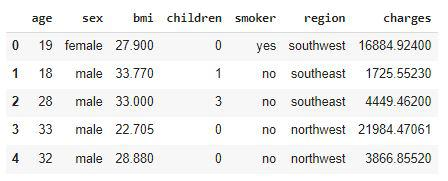First five rows of the dataset

Now, let’s check the shape of the dataset.

## Python3

 `df.shape`

Output:

`(1338, 7)`

This dataset contains 1338 data points with 6 independent features and 1 target feature.

## Python3

 `df.info()`

Output: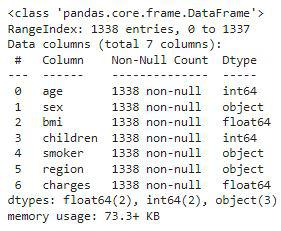Details about the columns of the dataset

From the above, we can see that the dataset contains 2 columns with float values 3 with categorical values and the rest contains integer values.

## Python3

 `df.describe()`

Output: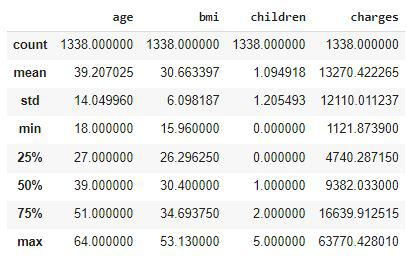Descriptive Statistical measures of the data

We can look at the descriptive statistical measures of the continuous data available in the dataset.

### Exploratory Data Analysis

EDA is an approach to analyzing the data using visual techniques. It is used to discover trends, and patterns, or to check assumptions with the help of statistical summaries and graphical representations. While performing the EDA of this dataset we will try to look at what is the relation between the independent features that is how one affects the other.

## Python3

 `df.isnull().``sum``()`

Output: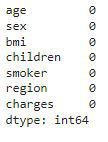Count of the null values column wise

So, here we can conclude that there are no null values in the dataset given.

## Python3

 `features ``=` `[``'sex'``, ``'smoker'``, ``'region'``]`` ` `plt.subplots(figsize``=``(``20``, ``10``))``for` `i, col ``in` `enumerate``(features):``    ``plt.subplot(``1``, ``3``, i ``+` `1``)`` ` `    ``x ``=` `df[col].value_counts()``    ``plt.pie(x.values,``            ``labels``=``x.index,``            ``autopct``=``'%1.1f%%'``)`` ` `plt.show()`

Output: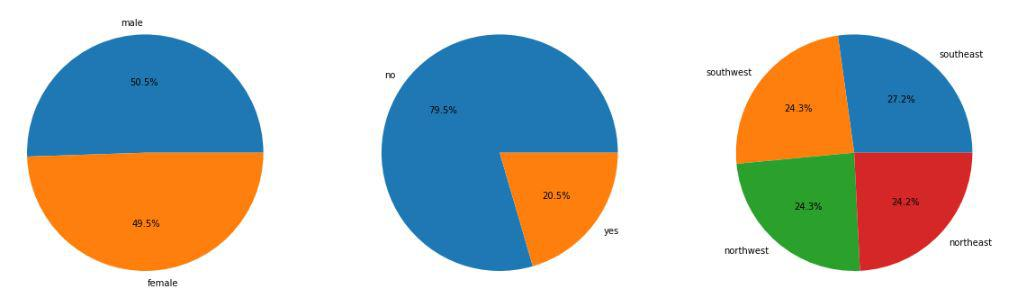Pie chart for the sex, smoker, and region column

The data provided to us is equally distributed among the sex and the region columns but in the smoker column, we can observe a ratio of 80:20.

## Python3

 `features ``=` `[``'sex'``, ``'children'``, ``'smoker'``, ``'region'``]`` ` `plt.subplots(figsize``=``(``20``, ``10``))``for` `i, col ``in` `enumerate``(features):``    ``plt.subplot(``2``, ``2``, i ``+` `1``)``    ``df.groupby(col).mean()[``'charges'``].plot.bar()``plt.show()`

Output: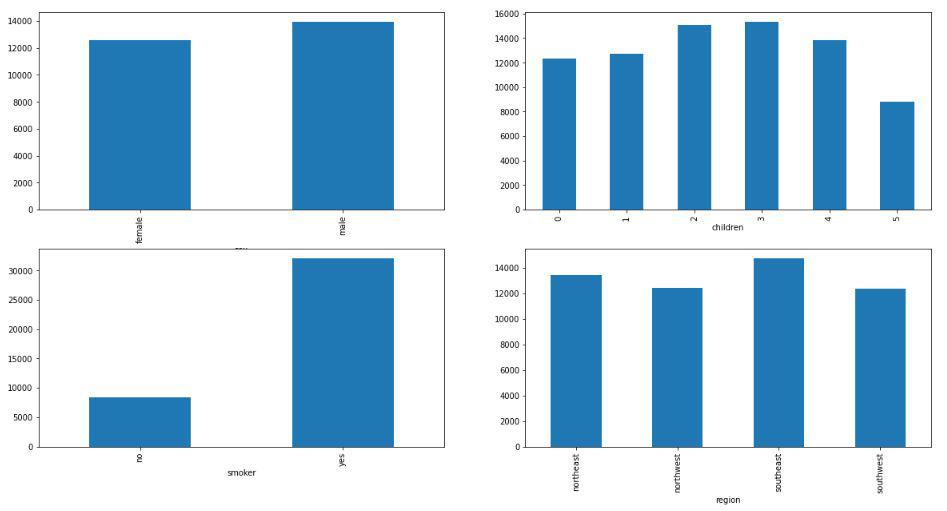Comparison between charges paid between different groups

Now let’s look at some of the observations which are shown in the above graphs:

• Charges are on the higher side for males as compared to females but the difference is not that much.
• Premium charged from the smoker is around thrice that which is charged from non-smokers.
• Charges are approximately the same in the given four regions.

## Python3

 `features ``=` `[``'age'``, ``'bmi'``]`` ` `plt.subplots(figsize``=``(``17``, ``7``))``for` `i, col ``in` `enumerate``(features):``    ``plt.subplot(``1``, ``2``, i ``+` `1``)``    ``sb.scatterplot(data``=``df, x``=``col,``                   ``y``=``'charges'``,``                   ``hue``=``'smoker'``)``plt.show()`

Output: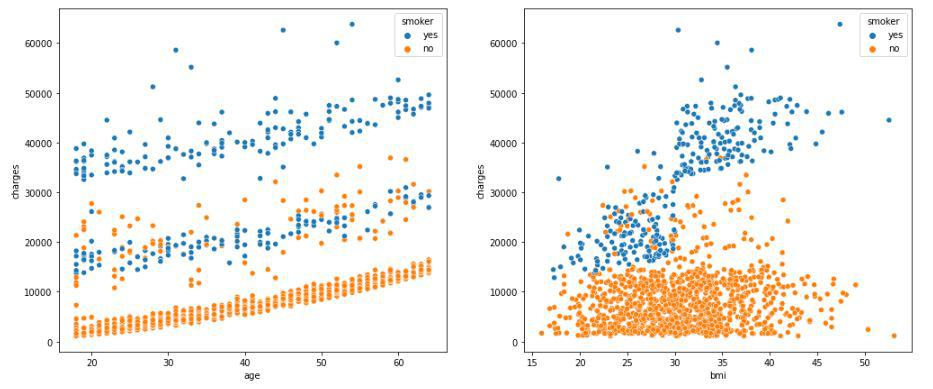Scatter plot of the charges paid v/s age and BMI respectively

A clear distinction can be observed here between the charges that smokers have to pay. Also here as well we can observe that as the age of a person increases premium prices goes up as well.

## Python3

 `features ``=` `[``'age'``, ``'bmi'``]`` ` `plt.subplots(figsize``=``(``17``, ``7``))``for` `i, col ``in` `enumerate``(features):``    ``plt.subplot(``1``, ``2``, i ``+` `1``)``    ``sb.distplot(df[col])``plt.show()`

Output: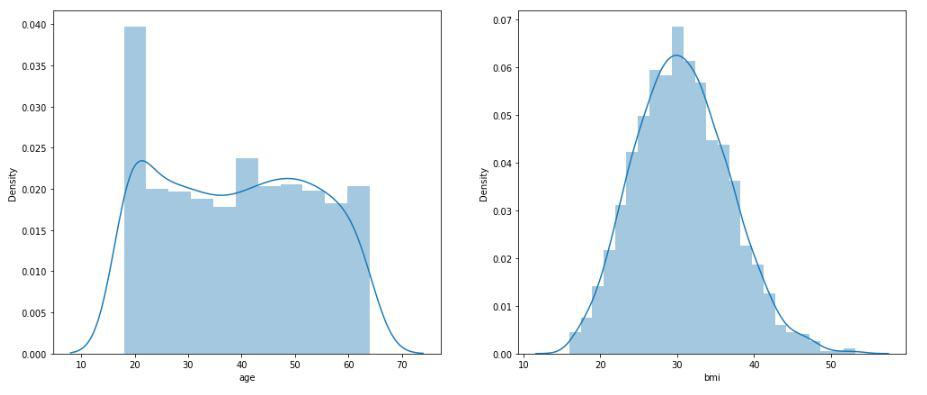Distribution plot of the age and BMI column

Data in both the age and BMI column approximately follow a Normal distribution which is a good point with respect to the model’s learning.

## Python3

 `features ``=` `[``'age'``, ``'bmi'``]`` ` `plt.subplots(figsize``=``(``17``, ``7``))``for` `i, col ``in` `enumerate``(features):``    ``plt.subplot(``1``, ``2``, i ``+` `1``)``    ``sb.boxplot(df[col])``plt.show()`

Output: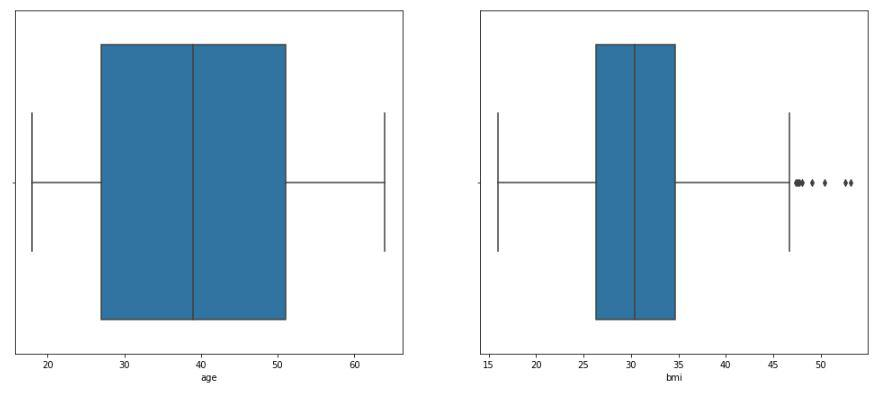Box plot of the age and BMI column

Ah! there are outliers in the BMI column of the given dataset. Let’s check how many rows of the dataset we will lose if we remove those outliers.

## Python3

 `df.shape, df[df[``'bmi'``]<``45``].shape`

Output:

`((1338, 7), (1318, 7))`

We will lose only 20 data points and the dataset will become free from any outliers so, we can do this sacrifice.

## Python3

 `df ``=` `df[df[``'bmi'``]<``45``]`

To analyze the correlation between the features of this dataset we must perform the LabelEncoding of the categorical columns.

## Python3

 `for` `col ``in` `df.columns:``    ``if` `df[col].dtype ``=``=` `object``:``        ``le ``=` `LabelEncoder()``        ``df[col] ``=` `le.fit_transform(df[col])`

Let’s draw a heatmap to analyze the correlation between the variables of the dataset.

## Python3

 `plt.figure(figsize``=``(``7``, ``7``))``sb.heatmap(df.corr() > ``0.8``,``           ``annot``=``True``,``           ``cbar``=``False``)``plt.show()`

Output: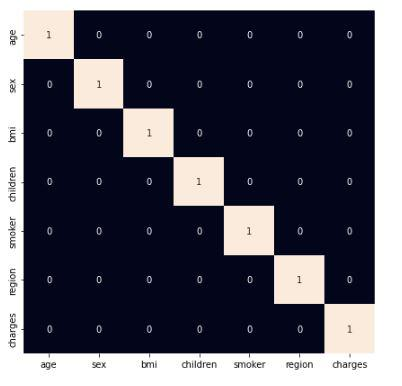Heatmap to analyze the correlation between features

From the above heatmap, it is certain that there are no highly correlated features in it.

### Model Development

There are so many state-of-the-art ML models available in academia but some model fits better to some problem while some fit better than other. So, to make this decision we split our data into training and validation data. Then we use the validation data to choose the model with the highest performance.

## Python3

 `features ``=` `df.drop(``'charges'``, axis``=``1``)``target ``=` `df[``'charges'``]`` ` `X_train, X_val,\``Y_train, Y_val ``=` `train_test_split(features, target,``                                  ``test_size``=``0.2``,``                                  ``random_state``=``22``)``X_train.shape, X_val.shape`

Output:

`((1054, 6), (264, 6))`

After dividing the data into training and validation data it is considered a better practice to achieve stable and fast training of the model.

## Python3

 `scaler ``=` `StandardScaler()``X_train ``=` `scaler.fit_transform(X_train)``X_val ``=` `scaler.transform(X_val)`

Now let’s train some state-of-the-art machine learning models on the training data and then use the validation data for choosing the best out of them for prediction.

## Python3

 `models ``=` `[LinearRegression(), XGBRegressor(),``          ``RandomForestRegressor(), AdaBoostRegressor(),``          ``Lasso(), Ridge()]`` ` `for` `i ``in` `range``(``6``):``    ``models[i].fit(X_train, Y_train)`` ` `    ``print``(f``'{models[i]} : '``)``    ``pred_train ``=` `models[i].predict(X_train)``    ``print``(``'Training Error : '``, mape(Y_train, pred_train))`` ` `    ``pred_val ``=` `models[i].predict(X_val)``    ``print``(``'Validation Error : '``, mape(Y_val, pred_val))``    ``print``()`

Output:

```LinearRegression() :
Training Error :  0.4188805629224119
Validation Error :  0.4504495878121591

XGBRegressor() :
Training Error :  0.2423798632758146
Validation Error :  0.2968607102447037

RandomForestRegressor() :
Training Error :  0.11874416980772626
Validation Error :  0.24169452488917798

Training Error :  0.6049583785013588
Validation Error :  0.620496923849186

Lasso() :
Training Error :  0.418841845707845
Validation Error :  0.45044188913851757

Ridge() :
Training Error :  0.4190871910460788
Validation Error :  0.45082076456283665```

Here we have used MAPE which is the Mean Absolute Percentage Error metric to evaluate the model’s performance. A 0.1 value of MAPE means that the error in the predictions from the actual value will be around 10%.

### Conclusion

Out of all the models RandomForestModel is giving the least value for the mean absolute percentage error this means predictions made by this model are close to the real values as compared to the other model.

The dataset we have used here was small still the conclusion we drew from them were quite similar to what is observed in the real-life scenario. If we would have a bigger dataset then we will be able to learn even deeper patterns in the relation between the independent features and the premium charged from the buyers.

My Personal Notes arrow_drop_up